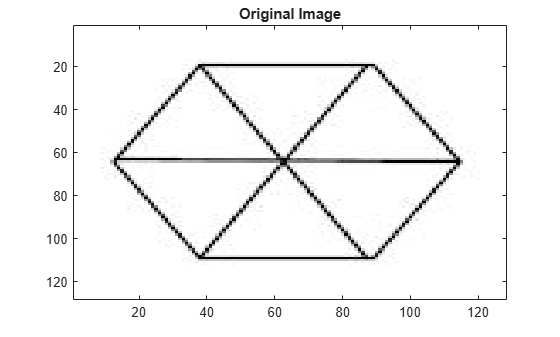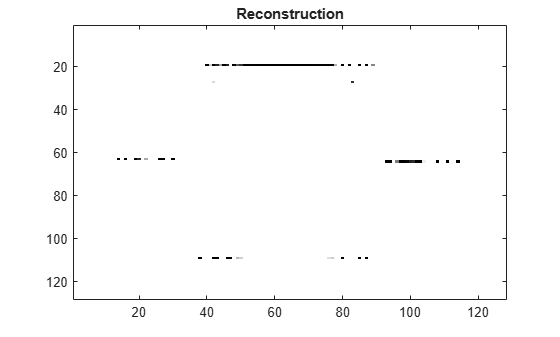# waverec2

Multilevel 2-D discrete wavelet transform reconstruction

## Syntax

``imrec = waverec2(c,s,wname)``
``imrec = waverec2(c,s,LoR,HiR)``
``imrec = waverec2(___,Name=Value)``

## Description

example

````imrec = waverec2(c,s,wname)` reconstructs the image, `imrec`, based on the multilevel discrete wavelet transform (DWT), `c`, and the bookkeeping matrix, `s`. The `waverec2` function uses the wavelet specified by `wname`.`imrec = waverec2(c,s,wname)` is equivalent to `imrec = appcoef2(c,s,wname,0)`.```
````imrec = waverec2(c,s,LoR,HiR)` reconstructs `imrec` using the specified lowpass (scaling) and highpass (wavelet) reconstruction filters `LoR` and `HiR`, respectively.```

example

````imrec = waverec2(___,Name=Value)` specifies options using one or more name-value arguments in addition to the input arguments in previous syntaxes. For example, to specify a gain of `0.25` for the lowpass (scaling) coefficients, set `LowpassGain` to `0.25`.```

## Examples

collapse all

`load woman`

Perform a level 2 wavelet decomposition of the image using the `sym4` wavelet.

```wv = 'sym4'; [c,s] = wavedec2(X,2,wv);```

Reconstruct the image from the wavelet decomposition structure.

`xrec = waverec2(c,s,wv);`

Check for perfect reconstruction.

`max(abs(X(:)-xrec(:)))`
```ans = 2.0989e-10 ```

Import an image of a hexagon.

```im = imread("hexagon.jpg"); imagesc(im) title("Original Image")```Obtain a one-level discrete wavelet decomposition of the image using the `bior4.4` wavelet.

```wv = "bior4.4"; lev = 1; [c,s] = wavedec2(im,lev,wv);```

Reconstruct the image without the finest-scale `HH` subband. Recall the `HH` subband corresponds to the diagonal details in the image.

```dgain = ones(lev,3); dgain(lev,3) = 0; imrec = waverec2(c,s,wv,DetailGain=dgain); imagesc(imrec) title("Reconstruction")```## Input Arguments

collapse all

Wavelet decomposition vector, specified as a real-valued vector. The vector `c` contains the approximation and detail coefficients organized by level. The bookkeeping matrix `s` is used to parse `c`. `c` and `s` are outputs of `wavedec2`.

Data Types: `double`

Bookkeeping matrix, specified as an integer-valued matrix. The matrix `s` contains the dimensions of the wavelet coefficients by level and is used to parse the wavelet decomposition vector `c`. `c` and `s` are outputs of `wavedec2`.

Data Types: `double`

Analyzing wavelet, specified as a character vector or string scalar.

Note

`waverec2` supports only Type 1 (orthogonal) or Type 2 (biorthogonal) wavelets. See `wfilters` for a list of orthogonal and biorthogonal wavelets.

Wavelet reconstruction filters, specified as a pair of even-length real-valued vectors. `LoR` is the lowpass (scaling) reconstruction filter, and `HiR` is the highpass (wavelet) reconstruction filter. The lengths of `LoR` and `HiR` must be equal. See `wfilters` for additional information.

Data Types: `double`

### Name-Value Arguments

Specify optional pairs of arguments as `Name1=Value1,...,NameN=ValueN`, where `Name` is the argument name and `Value` is the corresponding value. Name-value arguments must appear after other arguments, but the order of the pairs does not matter.

Example: `imrec = waverec2(c,s,Lo,Hi,LowpassGain=0.5)` sets the lowpass gain to `0.5`.

Since R2023a

Wavelet subband gains, specified as a real-valued lev-by-3 matrix, where lev is the level of the DWT. lev is equal to `size(s,1)-2`. `DetailGain` must have three columns, one for each of the wavelet subbands in the order `LH` (horizontal details), `HL` (vertical details), and `HH` (diagonal details). The elements of `DetailGain` are real numbers in the interval `[0,1]` and represent the gain the `waverec2` function applies to the coefficients in each subband.

Example: ```imrec = waverec2(c,s,Lo,Hi,DetailGain=[0 1 1; 0 1 1])``` sets gain of the `LH` subband at all levels to `0`.

Data Types: `double`

Since R2023a

Lowpass gain, specified as a real number in the interval `[0,1]`. The `waverec2` function applies the gain to the scaling coefficients for use in the reconstruction.

Example: `imrec = waverec2(c,s,"db2",Lowpassgain=0)` sets the gain for the lowpass (scaling) coefficients to `0`.

Data Types: `double`

## Version History

Introduced before R2006a

expand all